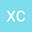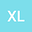On the P-formulation and the Split-Fraction-Formulation for the Generalized Pooling Problem
••Xin Cheng
Queen's University

Corresponding Author:[email protected]

Author Profile## Abstract

The generalized pooling problem (GPP) is a NP-hard problem for which the solution time for securing a global optimal solution heavily depends on the strength of the problem formulation. The existing GPP formulations use either quality variables (P-formulation and the variants) or split-fraction variables (SF-formulation and the variants) to model the material balance at the pools. This paper is the first attempt to develop theoretical results for comparing the strength of P-formulation and SF-formulation. It is found that, an enhanced version of P-formulation, called P+-formulation, is at least as strong as SF-formulation under mild conditions. Furthermore, P+-formulation becomes identical to P-formulation when the pooling network comprises only mixers and splitters. With additional conditions that are often satisfied at the root node, P-formulation is proved to be as least as strong as SF-formulation. The theoretical results are verified by the computational study of 23 problem instances.
Aug 2022Published in Computers & Chemical Engineering volume 164 on pages 107893. 10.1016/j.compchemeng.2022.107893